Predictive and Specialized Modeling > Fit Curve > Statistical Details for the Fit Curve Platform
Publication date: 08/13/2020

# Statistical Details for the Fit Curve Platform

## Model Formulas

Table 13.1 provides the formulas for the models on the Fit Curve red triangle menu.

Table 13.1 Fit Curve Model Formulas

Model

Formula

Polynomials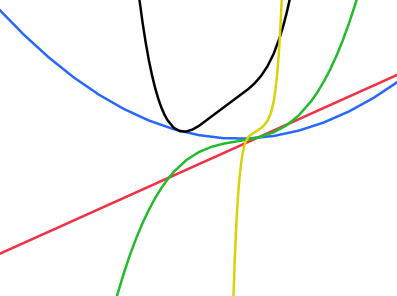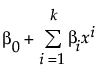where k is the order of the polynomial. These models can also be fit using the Fit Model and Fit Y by X platforms.

Logistic 2P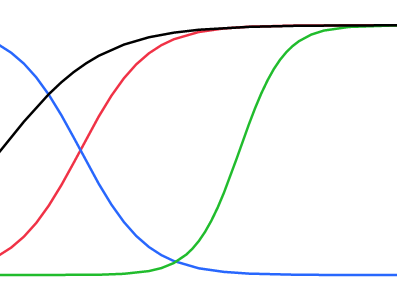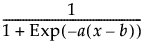a = Growth Rate

b = Inflection Point

Available only when all response values are between zero and one.

Logistic 3P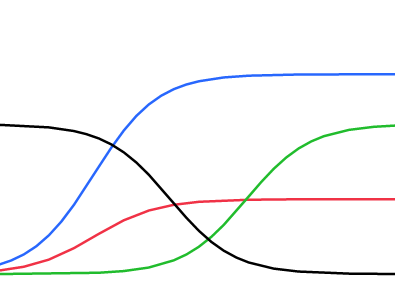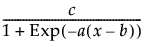a = Growth Rate

b = Inflection Point

c = Asymptote

Logistic 4P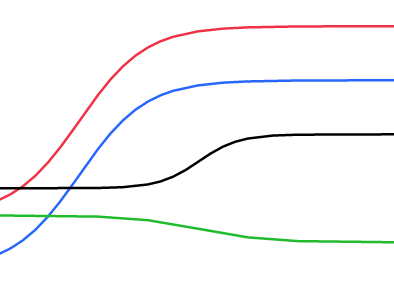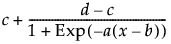a = Growth Rate

b = Inflection Point

c = Lower Asymptote

d = Upper Asymptote

Logistic 4P Rodbard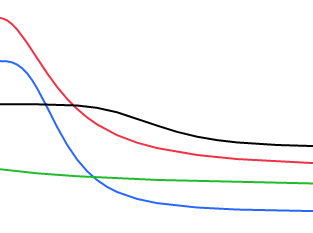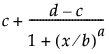a = Growth Rate

b = Inflection Point

c = Lower Asymptote

d = Upper Asymptote

Available only when the regressor values are positive.

Logistic 4P Hill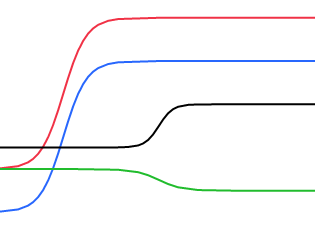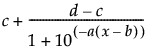a = Growth Rate

b = Inflection Point

c = Lower Asymptote

d = Upper Asymptote

Logistic 5P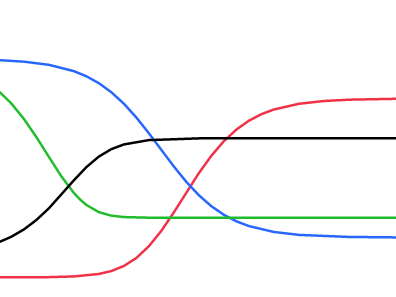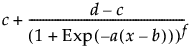a = Growth Rate

b = Inflection Point

c = Asymptote 1

d = Asymptote 2

f = Power

Probit 2P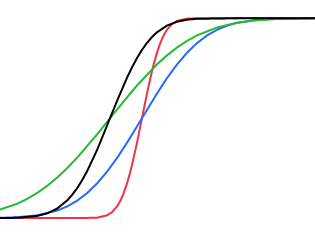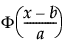a = Growth Rate

b = Inflection Point

Φ = Normal Distribution CDF

Available only when all response values are between zero and one.

Probit 4P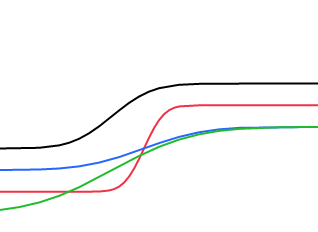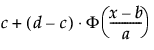a = Growth Rate

b = Inflection Point

c = Asymptote 1

d = Asymptote 2

Φ = Normal Distribution CDF

Gompertz 3P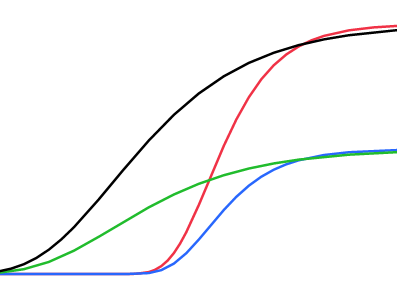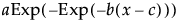a = Asymptote

b = Growth Rate

c = Inflection Point

Gompertz 4P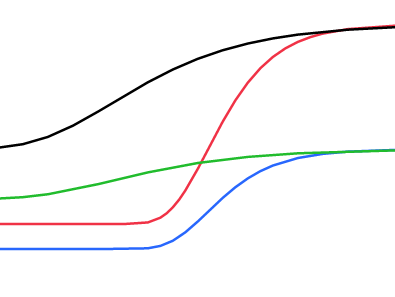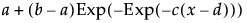a = Lower Asymptote

b = Upper Asymptote

c = Growth Rate

d = Inflection Point

Weibull Growth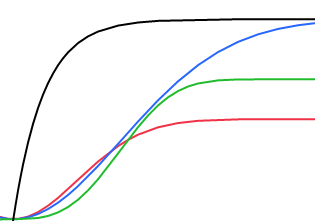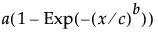a = Upper Asymptote

b = Growth Rate

c = Inflection Point

Available only when both the response values and regressor values are non-negative.

Exponential 2P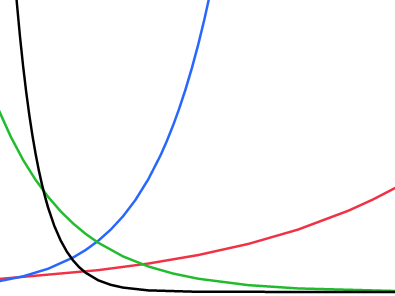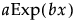a = Scale

b = Growth Rate

Exponential 3P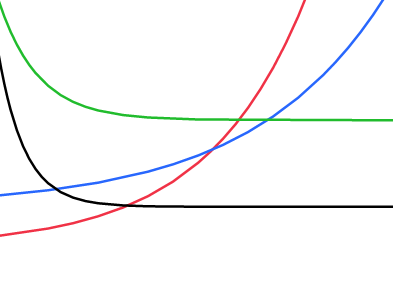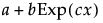a = Asymptote

b = Scale

c = Growth Rate

Biexponential 4P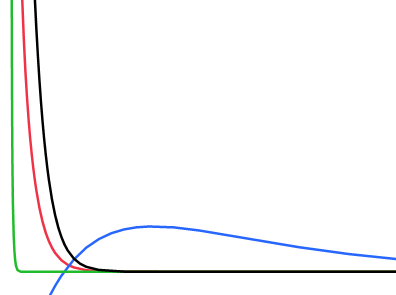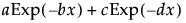a = Scale 1

b = Decay Rate 1

c = Scale 2

d = Decay Rate 2

Available only when the response values are positive.

Biexponential 5P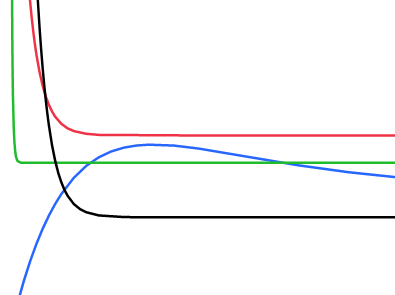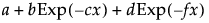a = Asymptote

b = Scale 1

c = Decay Rate 1

d = Scale 2

f = Decay Rate 2

Mechanistic Growth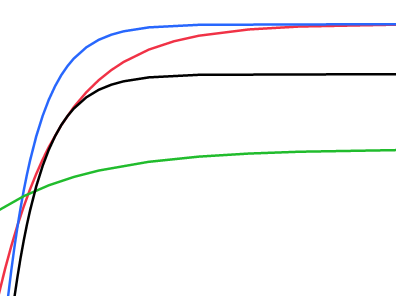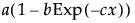a = Asymptote

b = Scale

c = Growth Rate

Cell Growth 4P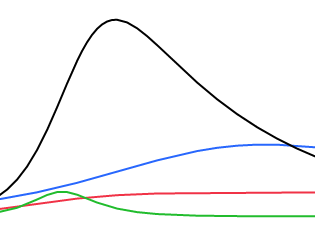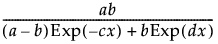a = Peak value if mortality rate, d, is zero

b = Response at time zero

c = Cell Division Rate

d = Cell Mortality Rate

Available only when the response values are positive and the regressor values are non-negative.

Gaussian Peak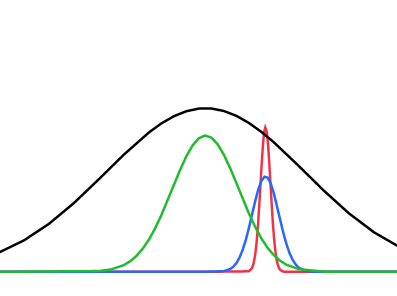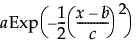a = Peak Value

b = Critical Point

c = Growth Rate

Lorentzian Peak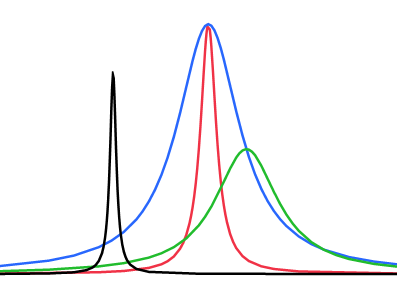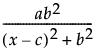a = Peak Value

b = Growth Rate

c = Critical Point

One Compartment Oral Dose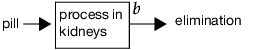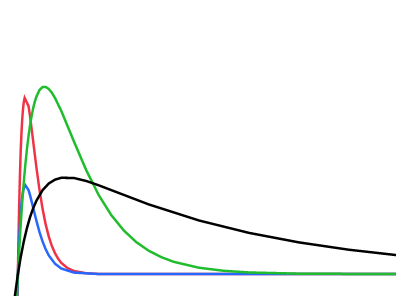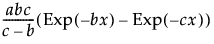a = Area Under Curve

b = Elimination Rate

c = Absorption Rate

Available only when the response values and the regressor values are all positive.

Two Compartment IV Bolus Dose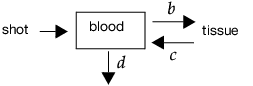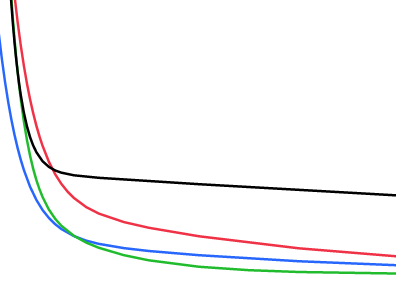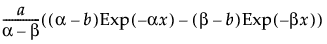α =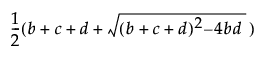β =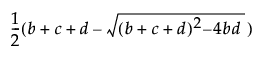a = Initial Concentration

b = Transfer Rate In

c = Transfer Rate Out

d = Elimination Rate

Available only when the response values and the regressor values are all positive.

Michaelis-Menten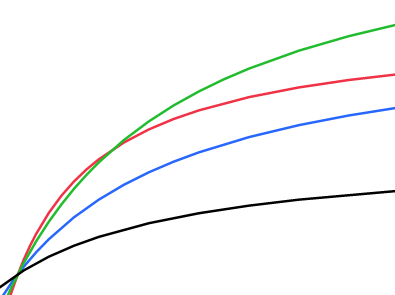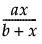a = Max Reaction Rate

b = Inverse Affinity

Available only when the response values and the regressor values are all positive.